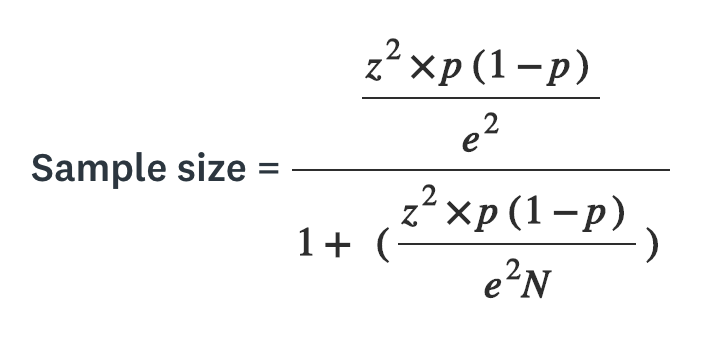# Google Data Analytics #15_Sample & Statistic

tpgk21·2022년 6월 30일
0

목록 보기
15/15## Sample Size

• a part of population that is representative of the population
• ex. when you have to use data that is just way too broad
• the goal is to get enough information from a small group within a population to make predictions or conclusions about the whole populationN = population size e = margin of error (percentage in decimal form) z = z-score

z-score is the number of standard deviations a given proportion is away from the mean

## Random Sample

• a way of selecting a sample from a population so that every possible type of the sample has an equal chance of being chosen
• this will reduce the sampling bias

## Statistical Power

• the probability of getting meaningful results from a test

## Hypothesis Testing

• a way to see if a survey or experiment has meaningful results

## Margin of Error

• maximum amount that the sample reuslts are expected to differ from those of the actual population

• to calculate the margin of error you need:

• population size
• sample size
• confidence level
• when executing A/B test, it is important to know about margin error

• if a returns 5% and b returns 3%, yet the margin of error is 2%, a overlaps the result of the b, therefore, the result is not statistically significant